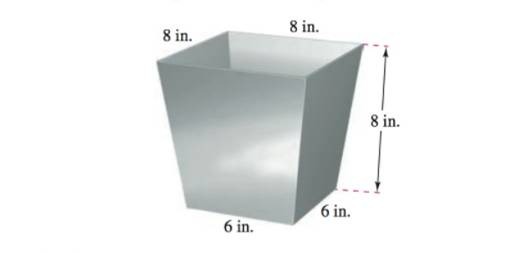Chapter 11.5, Problem 102E

Chapter
Section
Textbook Problem

# Mechanical Design The figure shows a chute at the top of a grain elevator of a combine that funnels the grain into a bin. Find the angle between two adjacent sides.To determine

To calculation: For the given adjacent sides, find the angle between two adjacent sides.

Explanation

Given:

The accompanying figure shows the chute at the top of a grain elevator of a combine that funnels the grain into a bin.

Formula used:

Angle between two planes is given by

cosθ=n1n2n1n2

Calculation:

Let us first, label the axes and the coordinates of edges of the provided mechanical design by using the dimensions shown in the design. Then,

Now here, point M=(8,8,8) as the x, y and z distances of point M from the axes is 8 units as provided in the design.

Also point S=(8,0,8) as the x and z distances of point S from the axes is 8 units as provided in the design and as the point is in xz plane, so its y-coordinate is zero.

Again point Q=(7,7,0) as the x and y distances of point Q from the axes is 7 units as the edge length of the square at the bottom surface is 6 units but the edge is starting from points x=1 and y=1.

Further point R=(0,8,8) as the height and length of vertex R from y axis and z axis respectively is 8 units as provided in design and as the point is in yz plane, so its x-coordinate is zero.

Now, the angle between the adjacent sides/planes of the design will be the angle between their normal vectors.

Consider two planes P1 and P2

To find the normal vector of a plane, three points on that plane is needed.

So, three points on plane P1 are Q, S and M.

Thus, the vectors MS and MQ in the plane P1 extending from point M to S and from M to Q are

MS=88,08,88=0,8,0

MQ=78,78,08=1,1,8

Now, the normal vector to plane P1 will be the cross product of MS and MQ

n1=MS×MQ=|ijk080118|=|8018|i|0018|j+|0811|k=64i+8j8k=64,8

### Still sussing out bartleby?

Check out a sample textbook solution.

See a sample solution

#### The Solution to Your Study Problems

Bartleby provides explanations to thousands of textbook problems written by our experts, many with advanced degrees!

Get Started